# Programming with Escher¶

This notebook is a collection of preliminary notes about a "code camp" (or a series of lectures) aimed at young students inspired by the fascinating Functional Geometry paper of Peter Henderson.

In such work the Square Limit woodcut by Maurits Cornelis Escher is reconstructed from a set of primitive graphical objects suitably composed by means of a functional language.

Here the approach will be somehow different: first of all because our recipients will be students new to computer science (instead of fellow researchers), but also because besides recalling the fundamental concepts of abstraction levels (and barriers), primitives and composition, present in the original paper, we will here also take the opportunity to introduce some (albeit to some extent elementary) considerations on algebra and geometry, programming and recursion (and perhaps discuss some implementation details).

This work is to be considered very preliminary, it is not yet structured in a series of lectures, nor it is worked out the level at which every topic is to be presented, according to the age (or previous knowledge) of the students. The language and detail level used here is intended for instructors and teachers, and the various topics will be listed as mere hints, not yet as a viable and ready to use syllabus.

As a last remark, before actually beginning with the notes, the code of this notebook is very loosely derived from previous "implementations" of Functional Geometry such as Shashi Gowda's Julia version and Micah Hahn's Hasjell version (containing the Bézier curve description of the Escher fish used here). I decided to rewrote such code in a Jupyter notebook written in Python 3, a simple and widespread language, to make it easier for instructors to adopt it.

The source notebook is available on GitHub (under GPL v3), feel free to use issues to point out errors, or to fork it to suggest edits.

## Square Limit and tiles¶

Looking at the original artwork it is evident that it is obtained by the repetition of a basic element (a fish) that is suitably oriented, scaled, and colored.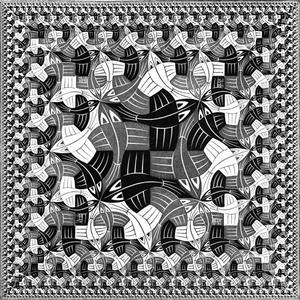This suggest to start our journey from a tile (a set of lines enclosed in the unit square), that is a drawing building block, that we will manipulate to obtain more complex drawings.

Note that, if one wants to follow an "unplugged" approach, tiles can be actually printed as objects so that students will be able to experiment with them in the physical world to better introduce themselves with the manipulations that will follow.

It is a good idea to start with an asymmetric tile, that will make it easier to grasp the effect of the transformations that will be presented.

f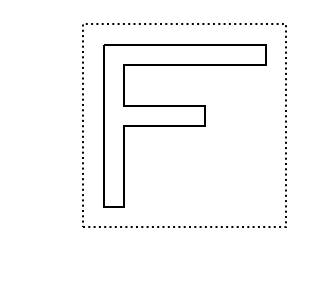Observe that the dotted line is not part of the tile but serves only as a hint for the tile boundary, moreover tiles are implemented in a way that the notebook automatically draws them (without the need of explicit drawing instructions).

## Transforming tiles¶

We are now ready to introduce some transformations, namely rot and flip that, respectively, rotate counterclockwise (by 90°) a tile, and flip it around its vertical center.

rot(f)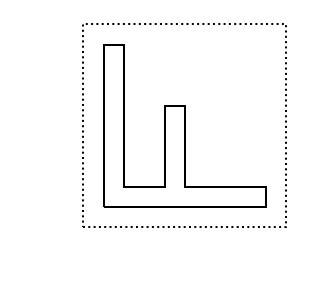Observe that the notation is the usual one for function application, that should be immediately clear even to students new to programming (but with a basic understanding of mathematical notation). Of course one can compose such transformations insomuch function can be composed, that is performed one on the result of another.

The first observation is that the order in which such transformation are performed can make a difference. Consider for instance

In :
flip(rot(f))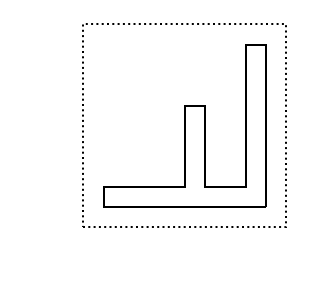In :
rot(flip(f))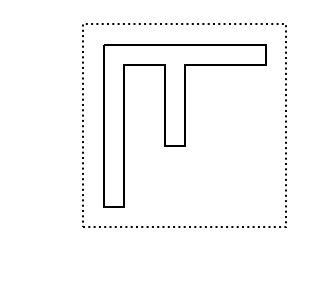The second observation is that some choices, that can seem at first restrictive, can become less of a limitation thanks to composition: we can obtain clockwise rotations by applying three counterclockwise rotations

In :
rot(rot(rot(f)))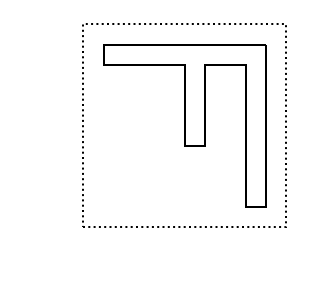this can stimulate a discussion about the expressiveness or completeness of a set of primitives with respect to an assigned set of tasks.

Then a few binary transformations (that is, transformation that operate on two tiles) can be introduced, such as: above, beside and over. The first two combine two tiles by juxtaposition (rescaling the final result so that it will again fit in a unit square), while the latter just lay one tile over another.

In :
beside(f, rot(f))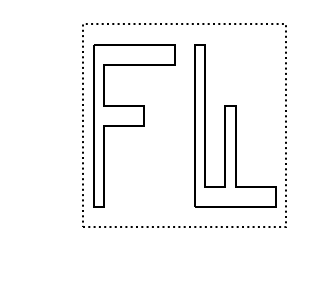In :
above(flip(f), f)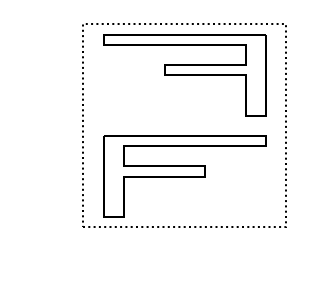Again one can observe that the order of the arguments is relevant, in the case of these two transformation, while it is not in the case of the latter.

In :
over(f, flip(f))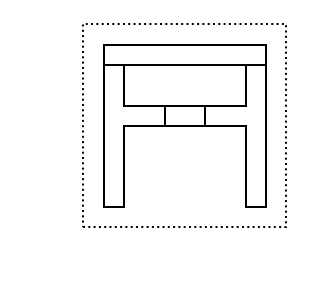## Basic algebraic facts about transformations¶

Such transformations can be also implemented as binary operators (thanks to Python ability to define classes that emulate numeric types); one can for instance use | and / respectively for beside and above, and + for over.

In :
f / ( f | f )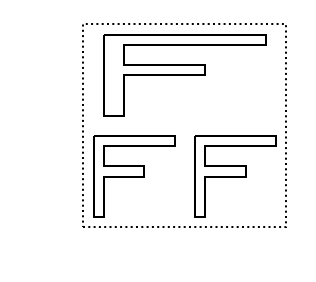This will allow to investigate (with a natural syntax) basic algebraic structures as (abelian or not) semigroups, and even monoids, once a blank tile is been introduced, as well as more simple concepts as associativity and commutativity,

In :
f + blank## Recursion¶

It can be quite natural to introduce functions, initially presented as a sort of macros, to build derived operators. For instance

In :
def quartet(p, q, r, s):
return above(beside(p, q), beside(r, s))


is a new transformation, defined in terms of the previous one.

In :
quartet(flip(rot(rot(rot(f)))), rot(rot(rot(f))), rot(f), flip(rot(f)))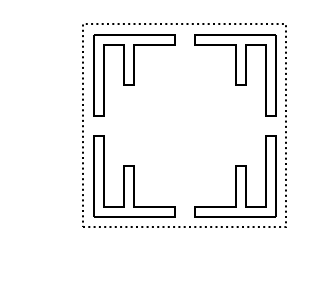Then recursion can be introduced quite naturally to build tiles having self-similar parts. Let's use a triangle to obtain a more pleasing result

In :
triangle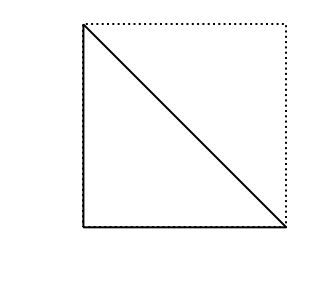Let's build a tile where the upper left quarter is a (rotated) triangle surrounded by three tiles similarly defined

In :
def rectri(n):
if n == 0:
return blank
else:
return quartet(rot(triangle), rectri(n - 1), rectri(n - 1), rectri(n - 1))


developing the first four levels gives

In :
rectri(4)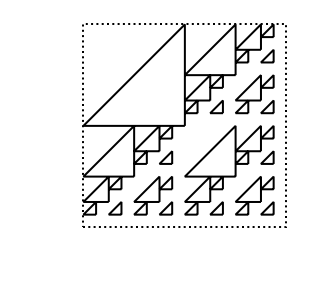Once can even push things further and show how to use of recursion instead of iteration, emphasizing how expressive a simple set of basic transformation, endowed with composition and recursion, can become.

## Extending the basic transformations¶

What if we want to write nonet, a version of quartet that puts together nine tiles in a $3\times 3$ arrangement? The given beside and above transformations are halving the width and height of the tiles they operate on, as it is easy to convince oneself, there is no way to use them to implement nonet.

To overcome such limitation, one can extend those transformations so that one can specify also the relative sizes of the combined tiles. For instance, in

In :
beside(flip(f), f, 2, 3)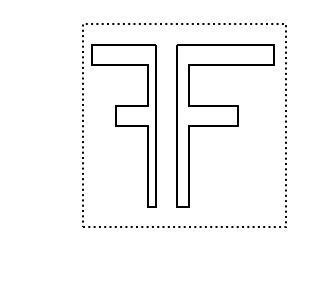the flipped f takes $2/5$ of the final tile, whereas f takes the other $3/5$. Using such extended transformations one can define

In :
def nonet(p, q, r, s, t, u, v, w, x):
return above(
beside(p, beside(q, r), 1, 2),
above(
beside(s, beside(t, u), 1, 2),
beside(v, beside(w, x), 1, 2),
),
1, 2
)


to obtain the desired result

In :
nonet(
f, f,     f,
f, blank, f,
f, f,     f
)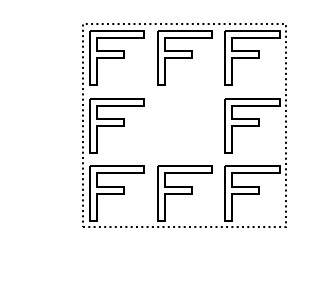of course, according to the way one decomposes the $3\times 3$ tile as a combination of two sub-tiles, there are many alternative ways to define nonet that students can experiment with.

Another possible approach will be to have above, beside (and over) accept a variable number of arguments (thanks to the way functions are defined and called in Python). In such case, otaining the nonet will be trivial.

## Decomposing the woodcut¶

The basic building block of the woodcut is a fish

In :
fish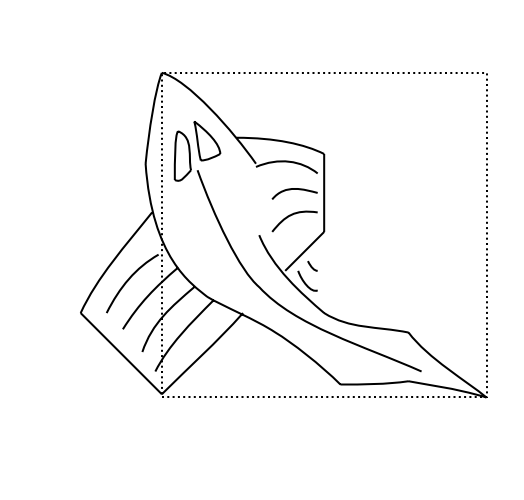even if it is not completely contained in a tile (the unit square), Escher choose (we'll discuss such magic in the following) a shape able to fit with his own rotation

In :
over(fish,rot(rot(fish)))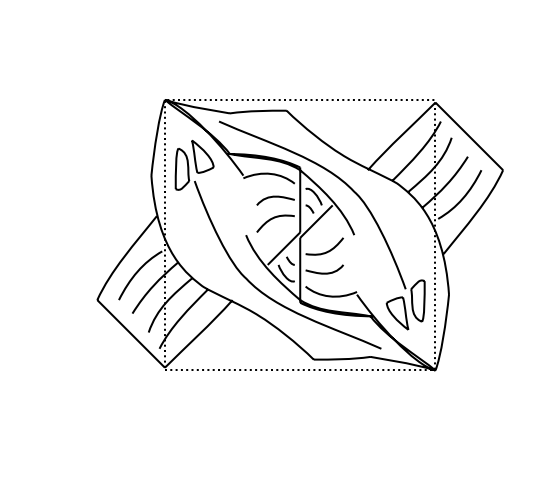But this is not the only magic. Let's define a new transformation that is a 45° rotation and a rescaling (by a $\sqrt 2$ factor) that, somehow, will "lift up" half tile; using it on triangle should clarify its definition

In :
rot45(triangle)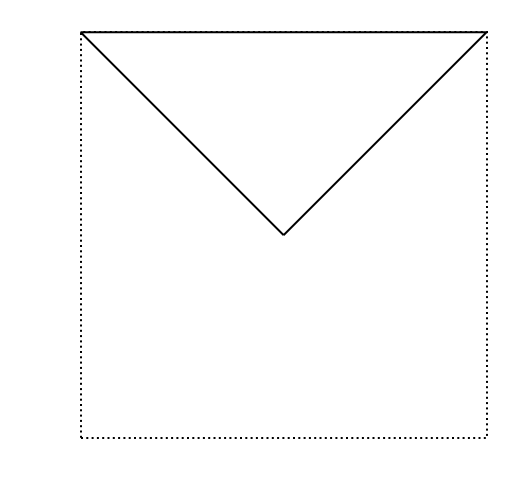Well, the fish is so magic that if we transform it with such new rotation and flip it

In :
smallfish = flip(rot45(fish))
smallfish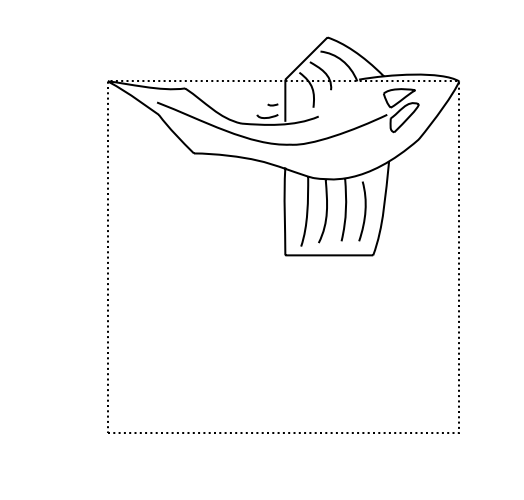we obtain a new tile that will fit with the original fish, even if rotated again

In :
t = over(fish, over(smallfish, rot(rot(rot(smallfish)))))
t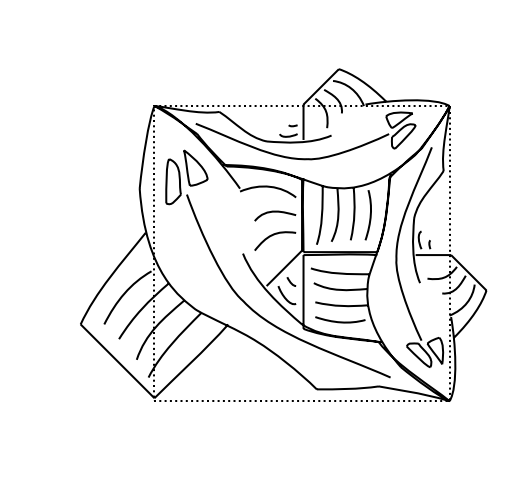and will moreover fit with itself however rotated

In :
u = over(over(over(smallfish, rot(smallfish)), rot(rot(smallfish))), rot(rot(rot(smallfish))))
u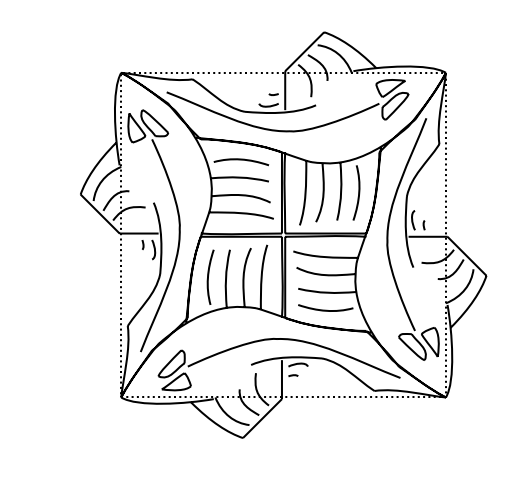The t and u tiles just defined are the building blocks of the woodcut; a recursive definition of the side, based just on t is given by

In :
def side(n):
if n == 0:
return blank
else:
return quartet(side(n-1), side(n-1), rot(t), t)


Expanding the first two levels gives

In :
side(2)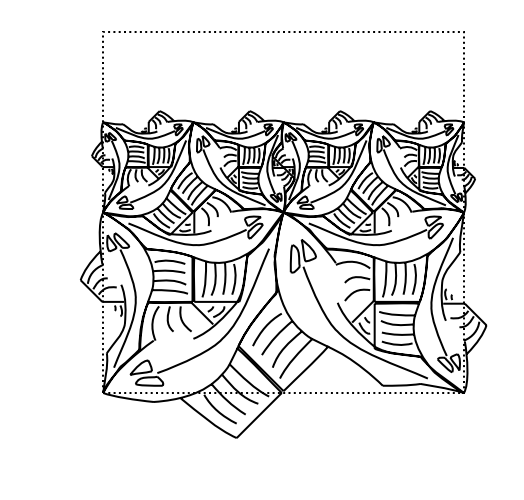Similarly, a recursive definition of the corner, based on the side and u is given by

In :
def corner(n):
if n == 0:
return blank
else:
return quartet(corner(n-1), side(n-1), rot(side(n-1)), u)


As before, the first two levels are

In :
corner(2)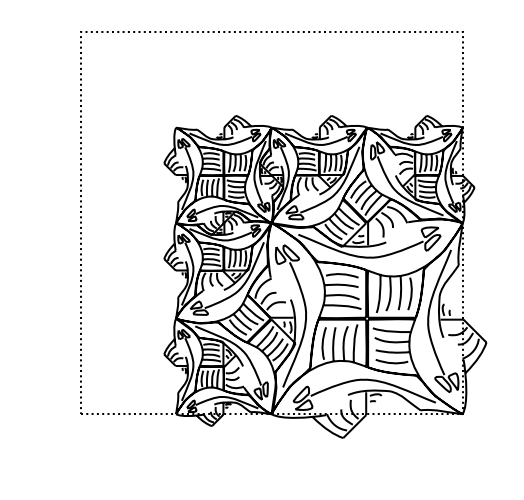We now can use a nonet to put together the (suitably rotated) sides and corners, as follows

In :
def squarelimit(n):
return nonet(
corner(n),
side(n),
rot(rot(rot(corner(n)))),
rot(side(n)),
u,
rot(rot(rot(side(n)))),
rot(corner(n)),
rot(rot(side(n))),
rot(rot(corner(n)))
)


Expanding the first three levels gives

In :
squarelimit(3)